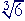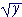S k i l l
i n
A L G E B R A

38

# LOGARITHMS

Definition

WHEN WE ARE GIVEN the base 2, for example, and exponent 3, then we can evaluate 23.

23 = 8.

Inversely, if we are given the base 2 and its power 8 --

2? = 8

-- then what is the exponent that will produce 8?

That exponent is called a logarithm.  We call the exponent 3 the logarithm of 8 with base 2.  We write

3 = log28.

The base 2 is written as a subscript.

3 is the exponent to which 2 must be raised to produce 8.

A logarithm is an exponent.

Since

104 = 10,000

then

log1010,000 = 4.

"The logarithm of 10,000 with base 10 is 4."

4 is the exponent to which 10 must be raised to produce 10,000.

"104 = 10,000" is called the exponential form.

"log1010,000 = 4" is called the logarithmic form.

Here is the definition:

logbx = n   means   bn = x.

That  base  with that  exponent  produces x.

Example 1.   Write in exponential form:   log232 = 5

 Example 2.   Write in logarithmic form:  4−2  = 116 .

Problem 1.   Which numbers have negative logarithms?

Do the problem yourself first!

Proper fractions.

Lesson 20 of Arithmetic

Example 3.   Evaluate  log81.

Answer.   8 to what exponent produces 1?

80 = 1.

log81 = 0.

We can observe that, in any base, the logarithm of 1 is 0.

logb1 = 0

Example 4.   Evaluate  log55.

Answer.   5 with what exponent will produce 5?   51 = 5.  Therefore,

log55 = 1.

In any base, the logarithm of the base itself is 1.

logbb = 1

Example 5.   log22m = ?

Answer.   2 with what exponent will produce 2m ?   m, obviously.

log22m = m.

The following is an important formal rule, valid for any base b:

logbbx = x

This rule embodies the very meaning of a logarithm.  x -- on the right -- is the exponent to which the base b must be raised  to produce bx.

 Example 6 .   Evaluate  log3 19 .
 Answer. 19 is equal to 3 with what exponent? 19 = 3−2.
 log3 19 = log33−2  =  −2.

Compare the previous rule.

Example 7.   log2 .25 = ?

Answer.   .25 = ¼ = 2−2.  Therefore,

log2 .25 = log22−2 = −2.

Example 8.   log3= ?

Answer.= 31/5.  (Definition of a rational exponent.)  Therefore,

log3= log331/5 = 1/5.

Problem 2.   Write each of the following in logarithmic form.

 a)    bn = x. logbx = n. b)    23 = 8. log28 = 3. c)    102 = 100. log10100 = 2. d)    5−2 =  1/25. log51/25 = −2.

Problem 3.   Write each of the following in exponential form.

 a)   logbx = n. bn = x. b)   log232 = 5. 25 = 32. c)   2 = log864. 82 = 64. d)   log61/36 = −2. 6−2 = 1/36.

Problem 4.   Evaluate the following.

 a)   log216 = 4 b)   log416 = 2 c)   log5125 = 3 d)   log81 = 0 e)   log88 = 1 f)   log101 = 0

Problem 5.   What number is n?

 a)   log10n = 3. 1000 b)   5 = log2n. 32 c)   log2n = 0. 1 d)   1 = log10n. 10
 e)   logn 116 = −2. 4 f)   logn 15 = −1. 5 g)   log2 132 = n. −5 h)   log2 12 = n. −1

Problem 6.   logbbx  =  x

Problem 7.   Evaluate the following.

 a)   log9 19 = log99−1 = −1
 b)   log9 181 = −2 c)   log2 14 = −2 d)   log2 18 = −3 e)   log2 116 = −4
 f)   log10.01 = −2 g)   log10.001 = −3 h)   log6= 1/3

Writein exponential form, and apply Problem 6.

 i)   logb= 3/4

Common logarithms

The system of common logarithms has 10 as its base.  When the base is not indicated:

log 100 = 2

then the system of common logarithms -- base 10 -- is implied.

Here are the powers of 10 and their logarithms:

 Powers of 10: 1   1000 1  100 110 1 10 100 1000 10,000 Logarithms: −3 −2 −1 0 1 2 3 4

Logarithms replace a geometric series with an arithmetic series.

Problem 8.

a)   log 105 = 5.  10 is the base.

b)   log 10n = n

c)   log 58 = 1.7634.   Therefore, 101.7634 = 58

1.7634 is the common logarithm of 58.  When 10 is raised to that exponent, 58 is produced.

Problem 9.   log (log x) = 1.  What number is x?

The log of what number is 1?  Since 10 is the base, log 10 = 1.

Therefore, log (log x) = 1 implies log x = 10.  And therefore since 10 is the base:

x = 1010 = 10,000,000,000

The three laws of logarithms

1.    logbxy  =  logbx  +  logby

"The logarithm of a product is equal to the sum
of the logarithms of each factor.
"

 2.    logb=  logbx  −  logby

"The logarithm of a quotient is equal to the logarithm of the numerator
minus the logarithm of the denominator.
"

3.    logb x n  =  n logbx

"The logarithm of a power of x is equal to the exponent of that power
times the logarithm of x.
"

For a proof of these laws, see Topic 20 of Precalculus.

 Example 9.    Apply the laws of logarithms to  log abc2 d 3 .

Answer.   According to the first two laws,

 log abc2 d 3 = log (abc2) − log d 3 = log a + log b + log c2 − log d 3 = log a + log b + 2 log c − 3 log d,

according to the third law.

The Answer above shows the complete theoretical steps.  In practice, however, it is not necessary to write the line

 log abc2 d 3 = log (abc2) − log d 3 .

The student should be able to go immediately to the next line --

 log abc2 d 3 = log a + log b + log c2 − log d 3

-- if not to the very last linelog abc2 d 3 = log a + log b + 2 log c − 3 log d.
 Example 10.   Apply the laws of logarithms to logz5 .
 Answer. logz5 =  log x  +  log−  log z5

Now,=  y½. (Lesson 29.)  Therefore, according to the third law,

 logz5 =  log x  +  ½ log y  −  5 log z.

Example 11.   Use the laws of logarithms to rewrite   log (sin x log x)

Solution.    This has the form  log aba = sin x,  b = log x.  Therefore,

log (sin x log x) = log sin x + log log x.

Example 12.   Use the laws of logarithms to rewrite  log.

Solution.

 log= log (x cos x)= ½ log (x cos x),   3rd Law = ½ (log x  +  log cos x),   1st Law.

Problem 10.   Use the laws of logarithms to rewrite the following.

 a)  log ab c = log a  +  log b  −  log c b)  log ab2 c4 = log a  +  2 log b  −  4 log c c)  logz = 1/3 log x  +  1/2 log y  −  log z
 d)   log (sin2x log x) = log sin2x  +  log log x = 2 log sin x  +  log log x
 e)   log= log (sin x cos x)1/2 = ½ log (sin x cos x) = ½ (log sin x  +  log cos x).

Example 13.   Given:  log 3 = .4771   Evaluate

a)   log 3000

Solution.  Write 3000 in scientific notation:

 log 3000 = log (3 × 103) = log 3 + log 103 = .4771 + 3 = 3.4771

b)   log .003

 Solution. log .003 = log (3 × 10−3) = log 3 + log 10−3 = .4771 − 3 = −2.5229

Problem 11.   Given:  log 6 = .7781   Use the laws of logarithms to evaluate the following.

 a)   log 600 = log (6 × 102) = log 6 + log 102 = .7781 + 2 = 2.7781
 b)   log 60 = log (6 × 10) = log 6 + log 10 = .7781 + 1 = 1.7781
 c)   log .06 = log (6 × 10−2) = log 6 + log 10−2 = .7781 − 2 = −1.2219

Example 14.   Given:  log 2 = .3010,  log 3 = .4771   Evaluate log 18.

Solution.   18 = 2· 32.  Therefore,

 log 18 = log (2· 32) = log 2 + log 32 = log 2 + 2 log 3 = .3010 + 2(.4771) = .3010 + .9542 = 1.2552

Problem 12.   Given:  log 2 = .3010    log 3 = .4771    log 5 = .6990

Use the laws of logarithms to find the following.

a)   log 6 = log 2 + log 3 = .7781

b)   log 15 = log 3 + log 5 = 1.1761

c)   log 4 = log 22 = 2 log 2 = .6020

d)   log 8 = log 2³ = 3 log 2 = .9030

e)   log 30 = log 3 + log 10 = 1.4771

f)   log 300 = log 3 + log 100 = 2.4771

g)   log 3000 = log 3 + log 1000 = 3.4771

h)   log 12 = log 3 + log 4 = 1.0791

 i)   log 35 = log 3 − log 5 = −.2219

j)   log= ½ log 3 = .2386

k)   log= ½ log 5 = .3495

 l)   log= 32 log 3 = .7157
 m)   log= 13 log 2 = .1003

n)   log= ½(log 2 − log 3) = −.0881

o)   log 1500 = log 3 + log 5 + log 100 = 3.1761

For the system of natural logarithms, see Topic 20 of Precalculus.

For logarithmic and exponential "functions," see Topic 21.Next Lesson:  Variation

Please make a donation to keep TheMathPage online.
Even \$1 will help.